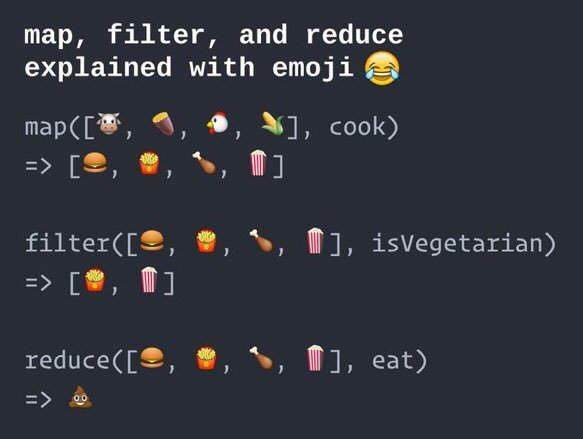# 试过不用循环语句撸代码吗？

### 示例1: 数组元素求和

const arrayOfNumbers = [17, -4, 3.2, 8.9, -1.3, 0, Math.PI];

#### 使用循环语句

let sum = 0;

arrayOfNumbers.forEach((number) => {
sum += number;
});

console.log(sum);

#### 不用循环语句

const sum = arrayOfNumbers.reduce((acc, number) =>
acc + number
);

console.log(sum);

const sum = ([number, ...rest]) => {
if (rest.length === 0) {
return number;
}
return number + sum(rest);
};

console.log(sum(arrayOfNumbers))

### 示例2: 将数组中的字符串拼接成句子

const dataArray = [0, 'H', {}, 'e', Math.PI, 'l', 'l', 2/9, 'o!'];

#### 使用循环语句

let string = '', i = 0;

while (dataArray[i] !== undefined) {
if (typeof dataArray[i] === 'string') {
string += dataArray[i];
}
i += 1;
}

console.log(string);

#### 不用循环语句

const string = dataArray.filter(e => typeof e === 'string').join('');

console.log(string);

### 示例3: 将数组元素变换为对象

const booksArray = [
'Clean Code',
'Code Complete',
'Introduction to Algorithms',
];

newArray = [
{ id: 1, title: 'Clean Code' },
{ id: 2, title: 'Code Complete' },
{ id: 3, title: 'Introduction to Algorithms' },
];

### 使用循环语句

const newArray = [];
let counter = 1;

for (let title of booksArray) {
newArray.push({
id: counter,
title,
});

counter += 1;
}

console.log(newArray);

### 不用循环语句

const newArray = booksArray.map((title, index) => ({
id: index + 1,
title
}));

console.log(newArray);

### 总结https://blog.fundebug.com/2017/11/13/write-javascript-without-loop/